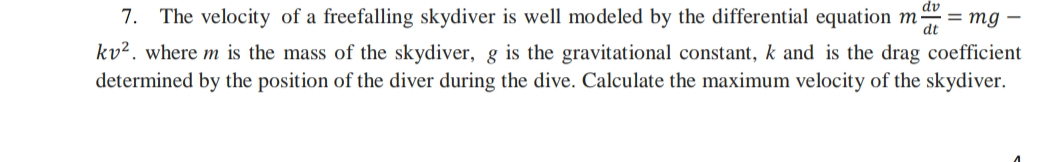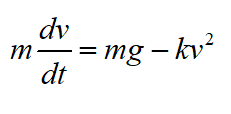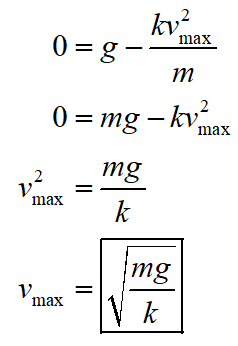# 7. The velocity of a freefalling skydiver is well modeled by the differential equation ma = mg –kv². where m is the mass of the skydiver, g is the gravitational constant, k and is the drag coefficientdetermined by the position of the diver during the dive. Calculate the maximum velocity of the skydiver.

Question
1 viewshelp_outlineImage Transcriptionclose7. The velocity of a freefalling skydiver is well modeled by the differential equation ma = mg – kv². where m is the mass of the skydiver, g is the gravitational constant, k and is the drag coefficient determined by the position of the diver during the dive. Calculate the maximum velocity of the skydiver. fullscreen
check_circle

Step 1

Consider the following equation.Step 2

Since the skydiver is falling freely. Therefore, the maximum velocity of the skydiver will be same as terminal velocity. Once the skydiver reaches to terminal velocity. The net force on the skydiver becomes zero.  Therefore,...

### Want to see the full answer?

See Solution

#### Want to see this answer and more?

Solutions are written by subject experts who are available 24/7. Questions are typically answered within 1 hour.*

See Solution
*Response times may vary by subject and question.
Tagged in

### Physics